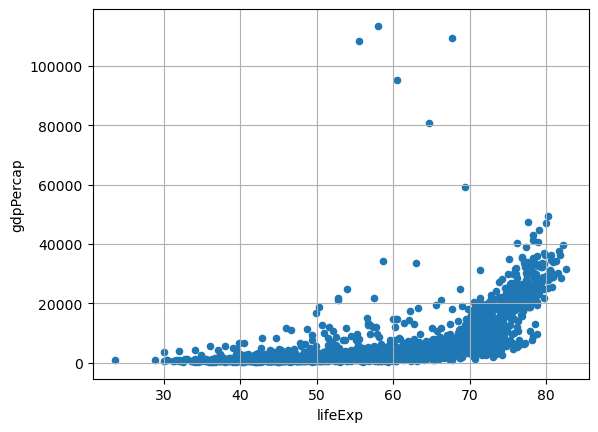A scatter plot is a graphical representation of data points in a dataset, where individual data points are plotted on a two-dimensional coordinate system. This type of plot allows us to visualize the relationship between two variables by showing how they are distributed across the plot.
Pandas, a powerful data manipulation library in Python, allows us to create scatter plots with ease. In this post, we will explore how to leverage `Pandas` to create simple scatter plots.

## Libraries

Pandas is a popular open-source Python library used for data manipulation and analysis. It provides data structures and functions that make working with structured data, such as tabular data (like `Excel` spreadsheets or `SQL` tables), easy and intuitive.

To install Pandas, you can use the following command in your command-line interface (such as `Terminal` or `Command Prompt`):

`pip install pandas`

Matplotlib functionalities have been integrated into the pandas library, facilitating their use with `dataframes` and `series`. For this reason, you might also need to import the matplotlib library when building charts with Pandas.

This also means that they use the same functions, and if you already know Matplotlib, you'll have no trouble learning plots with Pandas.

``````import pandas as pd
import matplotlib.pyplot as plt``````

## Dataset

In order to create graphics with Pandas, we need to use pandas objects: `Dataframes` and `Series`. A dataframe can be seen as an `Excel` table, and a series as a `column` in that table. This means that we must systematically convert our data into a format used by pandas.

Since scatter plots need quantitative variables, we will get the Gap Minder dataset using the `read_csv()` function. The data can be accessed using the url below.

``````url = 'https://raw.githubusercontent.com/holtzy/The-Python-Graph-Gallery/master/static/data/gapminderData.csv'

## Scatter plot with the scatter() function

Once we've opened our dataset, we'll now create the graph. The following displays the relation between the life expectancy and the gdp/capita using the `scatter()` function. This is probably one of the shortest ways to display a scatter plot in Python.

``````df.plot.scatter('lifeExp', # x-axis
'gdpPercap', # y-axis
grid=True, # Add a grid in the background
)
plt.show()``````## Scatter plot with the plot() function

We'll now look at how to create a scatter plot using the `plot()` function. This function is very general and therefore requires more arguments to be specified when it is called.

The main argument is `kind`. This specifies the type of chart we want (in our case it's `'scatter'`). For example, we could have put `'line'` for a line chart.

``````df.plot('lifeExp', # x-axis
'gdpPercap', # y-axis
kind='scatter', # type of chart
grid=True, # Add a grid in the background
)
plt.show()``````## Going further

This post explains how to create a simple scatter plot with pandas in 2 different ways.

For more examples of how to create or customize your plots with Pandas, see the pandas section. You may also be interested in how to customize your scatter plots with Matplotlib and Seaborn.

## Contact & Edit

👋 This document is a work by Yan Holtz. You can contribute on github, send me a feedback on twitter or subscribe to the newsletter to know when new examples are published! 🔥